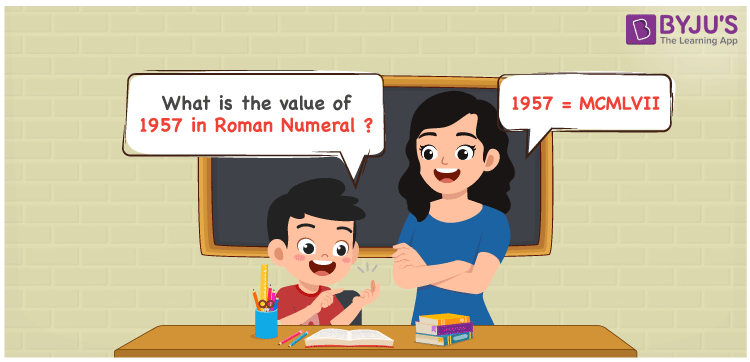# 1957 in Roman Numerals

1957 in Roman Numerals is MCMLVII. Roman numerals is an additive and subtractive system where letters are used to show certain numbers in the number system. A different combination of symbols are used to indicate the roman numerals that include the English alphabet. Therefore, 1957 can be written as MCMLVII in roman numerals.

 Number Roman Numeral 1957 MCMLVII## How to Write 1957 in Roman Numerals?

In order to write 1957 in Roman Numerals, it has to be written in expanded form, i.e.

1957 = 1000 + 900 + 50 + 7

1957 = M + CM + L + VII

1957 = MCMLVII

## Video Lesson on Roman Numerals## Frequently Asked Questions on 1957 in Roman Numerals

Q1

### Write 1957 in Roman Numerals.

In Roman Numerals, 1957 is written as MCMLVII.
Q2

### Write the numbers from 1950 to 1959 in roman numerals.

MCML = 1000 + 900 + 50 = 1950

MCMLI = 1000 + 900 + 50 + 1 = 1951

MCMLII = 1000 + 900 + 50 + 2 = 1952

MCMLIII = 1000 + 900 + 50 + 3 = 1953

MCMLIV = 1000 + 900 + 50 + 4 = 1954

MCMLV = 1000 + 900 + 50 + 5 = 1955

MCMLVI = 1000 + 900 + 50 + 6 = 1956

MCMLVII = 1000 + 900 + 50 + 7 = 1957

MCMLVIII = 1000 + 900 + 50 + 8 = 1958

MCMLIX = 1000 + 900 + 50 + 9 = 1959

Q3

### How to convert 1957 into Roman Numerals?

The conversion of 1957 into Roman Numerals includes breaking the number according to its place value.

Thousands = 1000 = M

Hundreds = 900 = CM

Tens = 50 = L

Ones = 7 = VII

Number = 1000 + 900 + 50 + 7 = M + CM + L + VII = MCMLVII